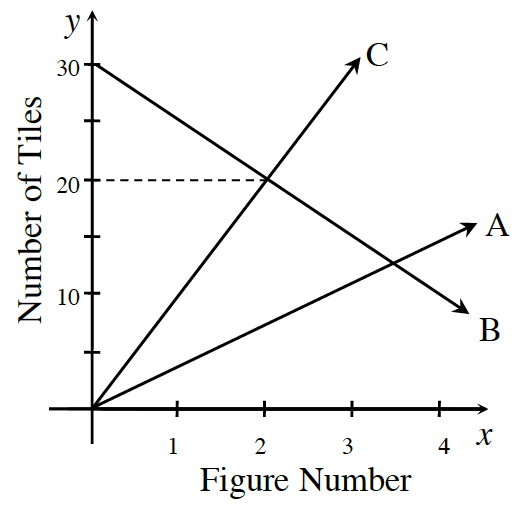### Home > CC3MN > Chapter 4 > Lesson 4.1.6 > Problem4-60

4-60.Examine the graph at right, which displays three tile patterns.

1. What do you know about Figure 0 for each of the three patterns?

What is the starting point for each pattern?

Figure $0$ has
Pattern A: $0$ tiles
Pattern B: $30$ tiles
Pattern C: $0$ tiles

2. Which pattern changes most quickly? How quickly does it change? Show how you know.

Find the growth of each pattern. For the pattern that changes the most quickly, the growth will be the greatest.

Pattern C grows the most quickly at $10$ tiles per figure. Its line is the steepest.

3. Which figure number has the same number of tiles in patterns B and C? Explain how you know.

Where two lines on the graph intersect, the number of tiles and the figure number are the same for those two patterns.

4. Write a rule for pattern B.

Use the starting point for pattern B you found in part (a) and the growth you found for pattern B in part (b).